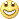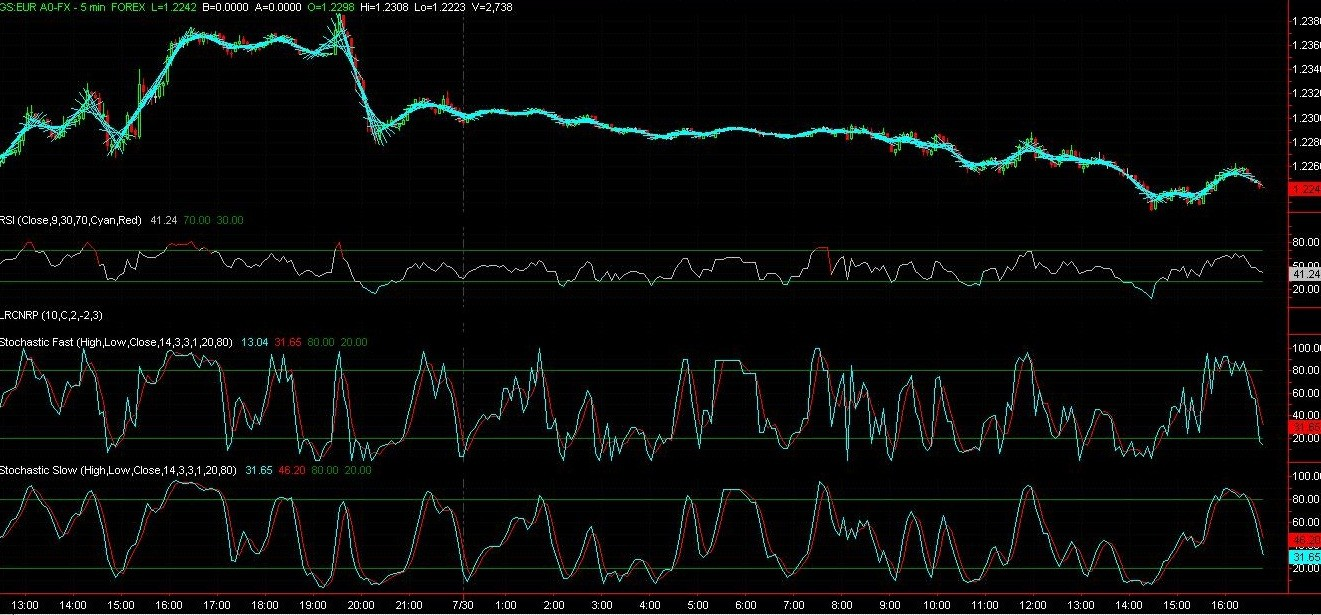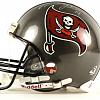# Linear Regression Indicator - page 69492

Hi Star821 and others who downloaded the last version, might want to download the correct version i posted 2 posts back i had made a high low version opposite breakout and got it mixed with the crossed version sorry for the hassle.5

Name of the indicator

MrTool Can you please tell me the name of the bar candles indicator thats in the elite section. Im a member, and I've been searching for it but I cant find it. Thanks in advance.30

mrtools:
Hi Star821 and others who downloaded the last version, might want to download the correct version i posted 2 posts back i had made a high low version opposite breakout and got it mixed with the crossed version sorry for the hassle.

Hi Mrtools. High low version is just what i want, I set high low band, when price cross up high band, i prepare to sell. when price croos down low band , i prepare to buy.9492

jorgel1234:
MrTool Can you please tell me the name of the bar candles indicator thats in the elite section. Im a member, and I've been searching for it but I cant find it. Thanks in advance.

Hi Jorgel,

They are called TTM_Ssa Bars, there is a version from here

https://www.mql5.com/en/forum/general373

LRC Non Refreshing

Hello, I would need some help to make a custom LRC Channel Indicator for MT4. I use it already in Tradestation and find it quite usefull. It looks like this, I'll explain below what it is :I got the Tradestation code from this video : h\$\$p://www.youtube.com/watch?v=peYbHALhyX8. It is basically a "bug linear regression channel indicator", non achieved, that draws on each bar but doesn't refresh and disappear for past bars. I like the way it looks like a moving average but with better spotting of reversal, so I use it in my trading. but as I trade in MT4, I would like to have equivalent in this platform.

Someone told me I should write a new code, based on drawing trendlines on each bar, but I really don't know how to deal with the linear regression channel calculation for the center bar.

Here is the tradestation code and a MT4 linear regression channel indicator (maybe it can be modified so that it does not refresh). Thanks a lot to those who are willing to help me.

linregrbuf.mq4

Inputs:

NumBars( 10 ),

Price( C ),

NumDevsUp( 2 ),

NumDevsDn( -2 ),

GraphOption( 3 );

Vars:

SumX( 0 ),

SumXX ( 0 ),

SumY( 0 ),

SumXY( 0 ),

m( 0 ),

_c( 0 ),

SDev( 0 ),

Left( 0 ),

Right( 0 ),

LowerLine( 0 ),

CenterLine( 0 ),

UpperLine( 0 ),

Flag( 0 );

Once

Begin

SumX = ( NumBars * ( NumBars + 1 )) / 2;

SumXX = ( Square(NumBars) * NumBars / 3) + (Square(NumBars) / 2 ) + NumBars / 6;

End;

For Value1 = 1 to NumBars

Begin

SumY = SumY + Price[Value1 - 1];

SumXY = SumXY + Value1 * Price[NumBars - Value1];

//Print(C[NumBars - Value1]);

End;

m = ( ( NumBars * SumXY ) - ( SumX * SumY ) ) / (NumBars * SumXX - Square( SumX) );

_c = ( ( SumY - ( m * SumX ) ) / NumBars );

// Left = m + _c;

// Right = NumBars * m + _c

Value2 = TL_New(D[NumBars-1],T[NumBars-1],m + _c,D,T,10*m + _c);

Print(D,T," Value2 ",Value2);

SumY = 0;

SumXY = 0;

Files:
untitled.jpg 196 kb
lrcnrp.png 152 kb
untitled_1.jpg 267 kb153997

It seems to be a series of linear regression lines (the middle lines of the linear regression channel)

It can be done but metarader might complain to it (since those are all going to be objects and metatrader does not "like" when there is a lot of objects on chart - it tends to slow down very much when there is too many object)

airquest:
Hello, I would need some help to make a custom LRC Channel Indicator for MT4. I use it already in Tradestation and find it quite usefull. It looks like this, I'll explain below what it is :I got the Tradestation code from this video : h\$\$p://www.youtube.com/watch?v=peYbHALhyX8. It is basically a "bug linear regression channel indicator", non achieved, that draws on each bar but doesn't refresh and disappear for past bars. I like the way it looks like a moving average but with better spotting of reversal, so I use it in my trading. but as I trade in MT4, I would like to have equivalent in this platform.

Someone told me I should write a new code, based on drawing trendlines on each bar, but I really don't know how to deal with the linear regression channel calculation for the center bar.

Here is the tradestation code and a MT4 linear regression channel indicator (maybe it can be modified so that it does not refresh). Thanks a lot to those who are willing to help me.

linregrbuf.mq4

Inputs:

NumBars( 10 ),

Price( C ),

NumDevsUp( 2 ),

NumDevsDn( -2 ),

GraphOption( 3 );

Vars:

SumX( 0 ),

SumXX ( 0 ),

SumY( 0 ),

SumXY( 0 ),

m( 0 ),

_c( 0 ),

SDev( 0 ),

Left( 0 ),

Right( 0 ),

LowerLine( 0 ),

CenterLine( 0 ),

UpperLine( 0 ),

Flag( 0 );

Once

Begin

SumX = ( NumBars * ( NumBars + 1 )) / 2;

SumXX = ( Square(NumBars) * NumBars / 3) + (Square(NumBars) / 2 ) + NumBars / 6;

End;

For Value1 = 1 to NumBars

Begin

SumY = SumY + Price[Value1 - 1];

SumXY = SumXY + Value1 * Price[NumBars - Value1];

//Print(C[NumBars - Value1]);

End;

m = ( ( NumBars * SumXY ) - ( SumX * SumY ) ) / (NumBars * SumXX - Square( SumX) );

_c = ( ( SumY - ( m * SumX ) ) / NumBars );

// Left = m + _c;

// Right = NumBars * m + _c

Value2 = TL_New(D[NumBars-1],T[NumBars-1],m + _c,D,T,10*m + _c);

Print(D,T," Value2 ",Value2);

SumY = 0;

SumXY = 0;4412

...

...looks like a Christmas Tree 2 me...373

It seems to be a series of linear regression lines (the middle lines of the linear regression channel) It can be done but metarader might complain to it (since those are all going to be objects and metatrader does not "like" when there is a lot of objects on chart - it tends to slow down very much when there is too many object)

@Pava : Yes, I know, looks a bit artistic.

@Mladen : Ok. I've got a couple of templates from traders that have a lot of objects (last one I've checked had 2484 objects). You're right it slows down a lot the system. So do you think it is possible to make the same indi with a limited number of objects drawn ? The number would be either fixed (between 100 and 1000, so that 100 to 1000 last bars have a trendline drawn) or customizable.373

It seems to be a series of linear regression lines (the middle lines of the linear regression channel) It can be done but metarader might complain to it (since those are all going to be objects and metatrader does not "like" when there is a lot of objects on chart - it tends to slow down very much when there is too many object)

Mladen, do you think you could help me to make this indi ? I really suck at coding. I know you must be very busy, there is no hurry at all, just would like to know if you're interested. I would understand if not. Thanks a lot.2047

Linear regression

Linear regression indicator with multiple levels

Files: